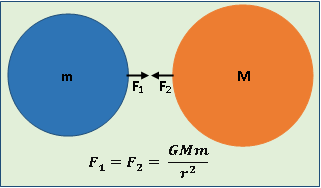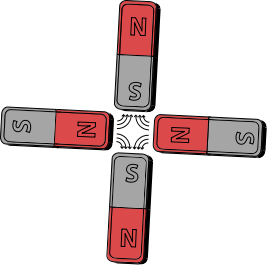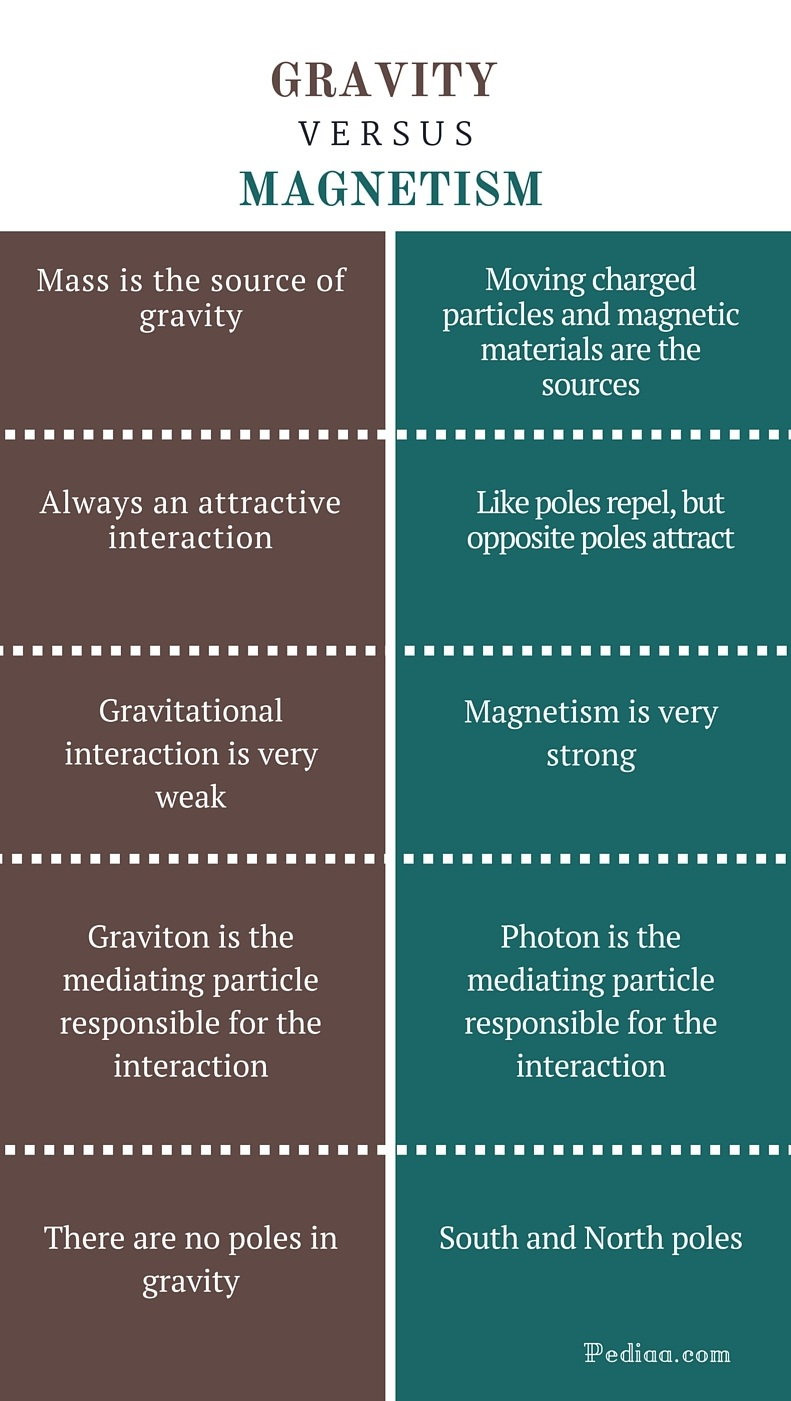# Difference Between Gravity and Magnetism

## Main Difference – Gravity vs Magnetism

Gravity and magnetism are two types of fundamental interactions in nature. Magnetism is a very strong interaction compared to gravity, which is the weakest interaction. Gravity is always an attractive interaction. In magnetism, both attractive and repulsive interactions are possible. The main difference between gravity and magnetism is that gravity is a consequence of space-time curvature caused by mass whereas magnetism is produced by moving charged particles or some materials. Gravity is a common property of both matter and anti-matter. However, magnetism is a special property of moving charged particles and magnetic materials. There are many other differences between gravity and magnetism. This article attempts to give you a better understanding of those differences.

## What is Gravity

In modern physics, gravity or gravitational interaction is one of the four fundamental interactions. Gravity isn’t a new concept; Several scientists and philosophers including Galileo Galilei and Aristotle attempted to explain and study the gravity. Eventually, great English scientist sir Isaac Newton developed a very successful theory of gravity. His theory is commonly referred to as “the Newton’s theory of gravitation” which states that every object with a mass attracts every other object through the gravitational force. According to his theory, the gravitational force exerted on an object due to mutual interaction with another object is directly proportional to the product of two masses and inversely proportional to the square of the distance between the two objects. This is usually expressed as F = GMm/r2 where F is the gravitational force, G is the universal gravitational constant, r is the distance between the two objects, and M and m are the masses of the two objects. Newton thought that his theory was a universal theory which could be used to explain any gravitational interaction in the universe. However, in the 20th century, some astronomical phenomena were observed that cannot be explained using the Newton’s theory of gravitation.

Newton’s theory of gravitation isn’t a very accurate universal theory. Its solutions notably deviate from the absolute values, when it is used to solve high gravity problems. However, Newton’s theory is sufficiently accurate enough to be used in low gravity phenomena.

In 1916, the Einstein theory of general relativity opened a new era in physics. According to his theory, the gravity isn’t a force but a consequence of space-time curvature caused by matter. Gravitational interaction is the weakest interaction out of the four fundamental interactions. It isn’t effective over short distances. The mediating particle of the gravitational interaction is the massless particle called “graviton.”

Einstein theory of gravity is very successful and can even be used to explain very complex gravitational phenomena in the universe. Anyhow, the Einstein theory of gravity is approximated to Newton’s theory when dealing with law gravity applications.## What is Magnetism

Magnetism is a physical phenomenon caused by some materials and moving charged particles. Magnetism is simply, the interaction of some materials and moving charged particles through the electromagnetic interaction. So, the mediating particle in magnetism is the photon.

Magnetism has two different types of sources. They are moving charged particles and magnetic materials. The most common moving charged particles are electrons. An electric current is a flood of moving electrons. So, an electric current can produce a magnetic field around it. This property is used in many applications such as electromagnets. An electromagnet is a magnet that produces a magnetic field by the flow of an electric current through a coil.

Materials that produce magnetic fields are called magnetic materials. Normally, electrons of an atom are paired up: one electron with spin up and the other electron with spin down. So, the net magnetic effect of the pair cancels out. But, in some materials, atoms contain unpaired electrons. So, those unpaired electrons can produce magnetism. Usually, magnetic materials are classified into three groups depending on their magnetic properties (How they response to external magnetic fields, their intrinsic magnetic moments). They are diamagnetic, paramagnetic and ferromagnetic materials. Diamagnetic materials scarcely repel strong magnetic fields whereas paramagnetic materials scarcely attract. But, ferromagnetic materials such as Iron are strongly attracted to external magnetic fields. Some materials such as Nickel and Cobalt can retain their magnetism for a long time once they are magnetized. So, they are known as permanent magnets.## Difference Between Gravity and Magnetism

### Sources:

Gravity: Mass is the source of gravity.

Magnetism: Moving charged particles and magnetic materials are the sources of magnetism.

### Nature of the Interaction

Gravity: Gravity is always an attractive interaction.

Magnetism: Like poles (South – South poles or North – North poles) repel. But opposite poles (South -North poles) attract.

### Relative Strength of the Interaction:

Gravity: Gravitational interaction is very weak.

Magnetism: Magnetism is very strong compared to gravitational interaction.

### Mediating Particle:

Gravity: Graviton is the mediating particle responsible for the interaction.

Magnetism: Photon is the mediating particle responsible for the interaction.

### Poles:

Gravity: There are no poles in gravity.

Magnetism: South and North poles.Image Courtesy:

“A magnetic quadrupole” by K. Aainsqatsi at English Wikipedia – Originally uploaded to the English Language Wikipedia, (Public Domain) via Commons Wikimedia